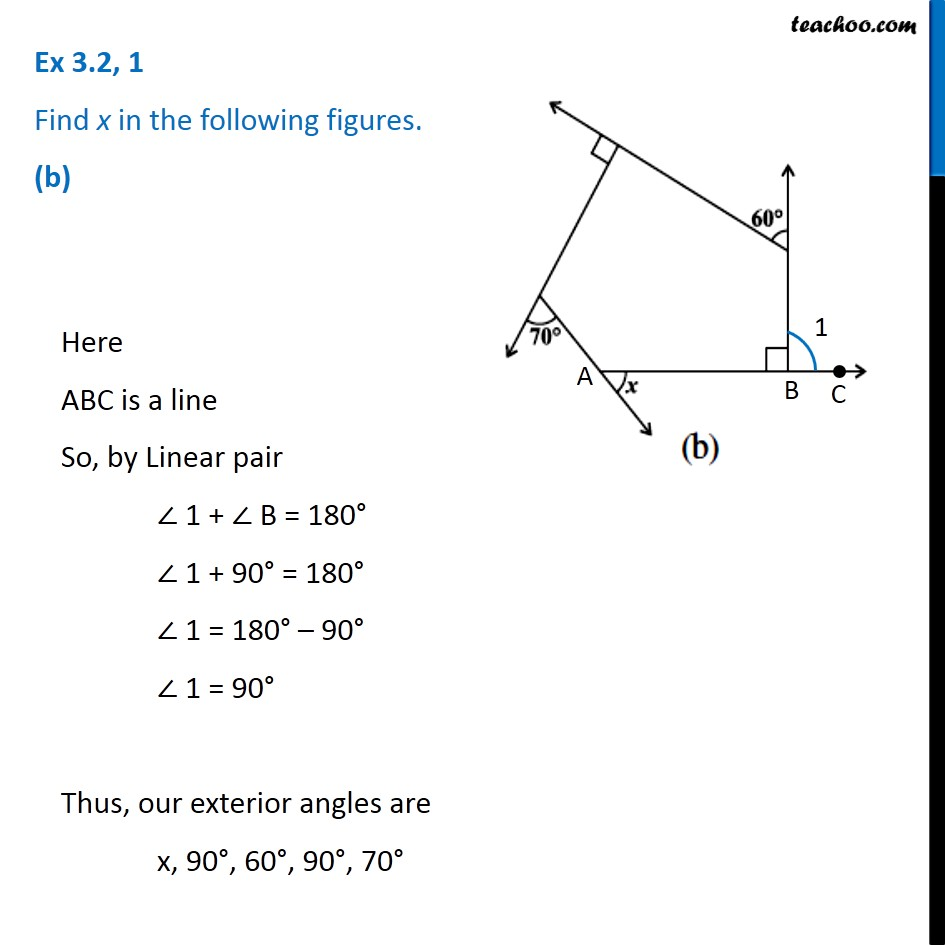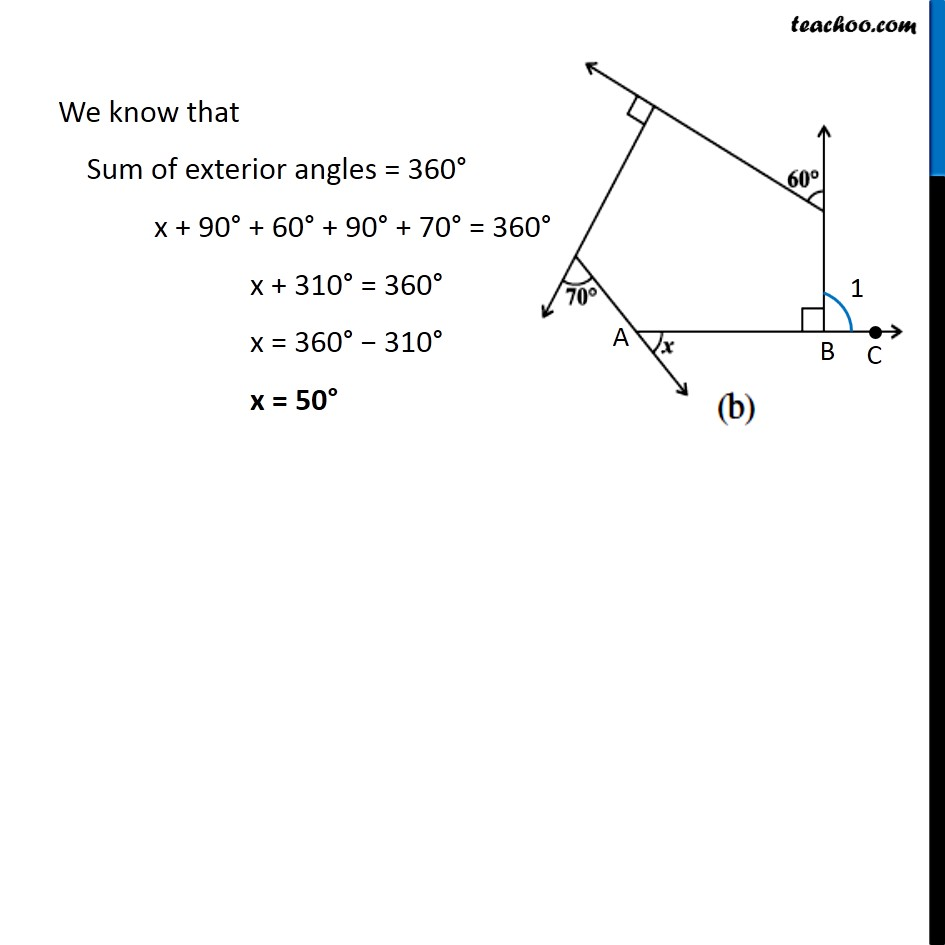Ex 3.2

Chapter 3 Class 8 Understanding Quadrilaterals
Serial order wiseLearn in your speed, with individual attention - Teachoo Maths 1-on-1 Class

### Transcript

Ex 3.2, 1 Find x in the following figures. (b) Here ABC is a line So, by Linear pair ∠ 1 + ∠ B = 180° ∠ 1 + 90° = 180° ∠ 1 = 180° – 90° ∠ 1 = 90° Thus, our exterior angles are x, 90°, 60°, 90°, 70° We know that Sum of exterior angles = 360° x + 90° + 60° + 90° + 70° = 360° x + 310° = 360° x = 360° − 310° x = 50°